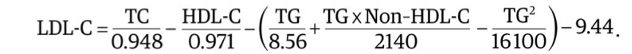top of page
Search

# A New Equation for Calculation of LDL-C in Patients with Normolipidemia and/or Hypertriglyceridemia

Author: Qian Sun, PhD, Assistant Professor of Pathology, William Beaumont School of Medicine, Oakland UniversityLow-density lipoprotein cholesterol (LDL-C), a key cardiovascular disease marker, is often estimated by the Friedewald or Martin equation. However, the calculated LDL-C in this way is less accurate in patients with low LDL-C levels or hypertriglyceridemia (triglyceride [TG] levels ≥400 mg/dL). Dr. Qian Sun and her colleagues developed a new equation to calculate LDL-C for these patients based on a large set of blood lipid test results (1).

The study used a de-identified dataset from 8,656 fasting patients tested on multiple occasions at the National Institutes of Health Clinical Center between January 1, 1976, and June 2, 1999. The dataset included results of β-quantification for LDL-C, a reference test method. Test results of LDL-C (n = 18715) and other lipids were randomly divided into equally sized training and validation datasets. Using TG and non-high-density lipoprotein cholesterol as independent variables, multiple least squares regression was used to establish an equation for very low-density lipoprotein cholesterol [VLDL-C] (Equation 1). Based on Equation 1, another equation for LDL-C was established (Equation 2).Equation 1Equation 2

The New equations were tested against the internal validation dataset, as well as multiple external datasets of either β-quantification LDL-C results or direct LDL-C test results.

Compared with β-quantification, the new equation was more accurate than other LDL-C equations: slope, 0.964; root mean square error (RMSE) = 15.2 mg/dL; R2 = 0.9648; vs Friedewald equation: slope, 1.056; RMSE = 32 mg/dL; R2 = 0.8808; vs Martin equation: slope, 0.945; RMSE = 25.7 mg/dL; R2 = 0.9022. Particularly, for patients with hypertriglyceridemia, compared with β-quantification, the new equation: mean absolute difference  (MAD) = 24.9 mg/dL; vs Friedewald equation: MAD = 56.4 mg/dL; vs Martin equation: MAD = 44.8 mg/dL. The new equation was able to achieve same-level accuracy when calculating the LDL-C level with TG levels up to 800 mg/dL as the Friedewald equation did with TG levels less than 400 mg/dL. It could result in 35% fewer misclassifications, which were defined as patients with hypertriglyceridemia (TG levels, 400-800 mg/dL) categorized into different LDL-C treatment groups.

The new equation can be readily implemented by clinical laboratories with no additional costs compared with the standard lipid panel. It will allow for more accurate calculation of LDL-C level in patients with low LDL-C levels and/or hypertriglyceridemia (TG levels, ≤800 mg/dL) and thus should improve the use of LDL-C level in cardiovascular disease risk management. So far, the major reference laboratories LabCorp and Mayo Clinic Lab have adopted this new equation.

Recognizing the limitation of the conventional Friedewald equation in hypertriglyceridemic individuals and its inaccuracy in the low range of LDL-C, cardiology societies in both the US and Europe have recommended alternative LDL-C calculations (2). In addition to the equation introduced in this article, the Martin equation that can be licensed from John Hopkins uses table search to report estimated LDL-C derived from ultracentrifugation measurement (3). Both can be considered to fill in the vacancy left behind after the future retirement of the Friedewald equation.Acknowledgements

We thank Dr. Jing Cao for revising the article.

References

1. Sampson M, Ling C, Sun Q, Harb R, Ashmaig M, Warnick R, et al. A New Equation for Calculation of Low-Density Lipoprotein Cholesterol in Patients With Normolipidemia and/or Hypertriglyceridemia. JAMA Cardiol 2020;5:540-8.

2. Langlois MR, Nordestgaard BG, Langsted A, Chapman MJ, Aakre KM, Baum H, et al. Quantifying atherogenic lipoproteins for lipid-lowering strategies: consensus-based recommendations from EAS and EFLM. Clin Chem Lab Med 2020;58:496-517.

3. Sajja A, Park J, Sathiyakumar V, Varghese B, Pallazola VA, Marvel FA, et al. Comparison of Methods to Estimate Low-Density Lipoprotein Cholesterol in Patients With High Triglyceride Levels. JAMA Netw Open 2021;4:e2128817.